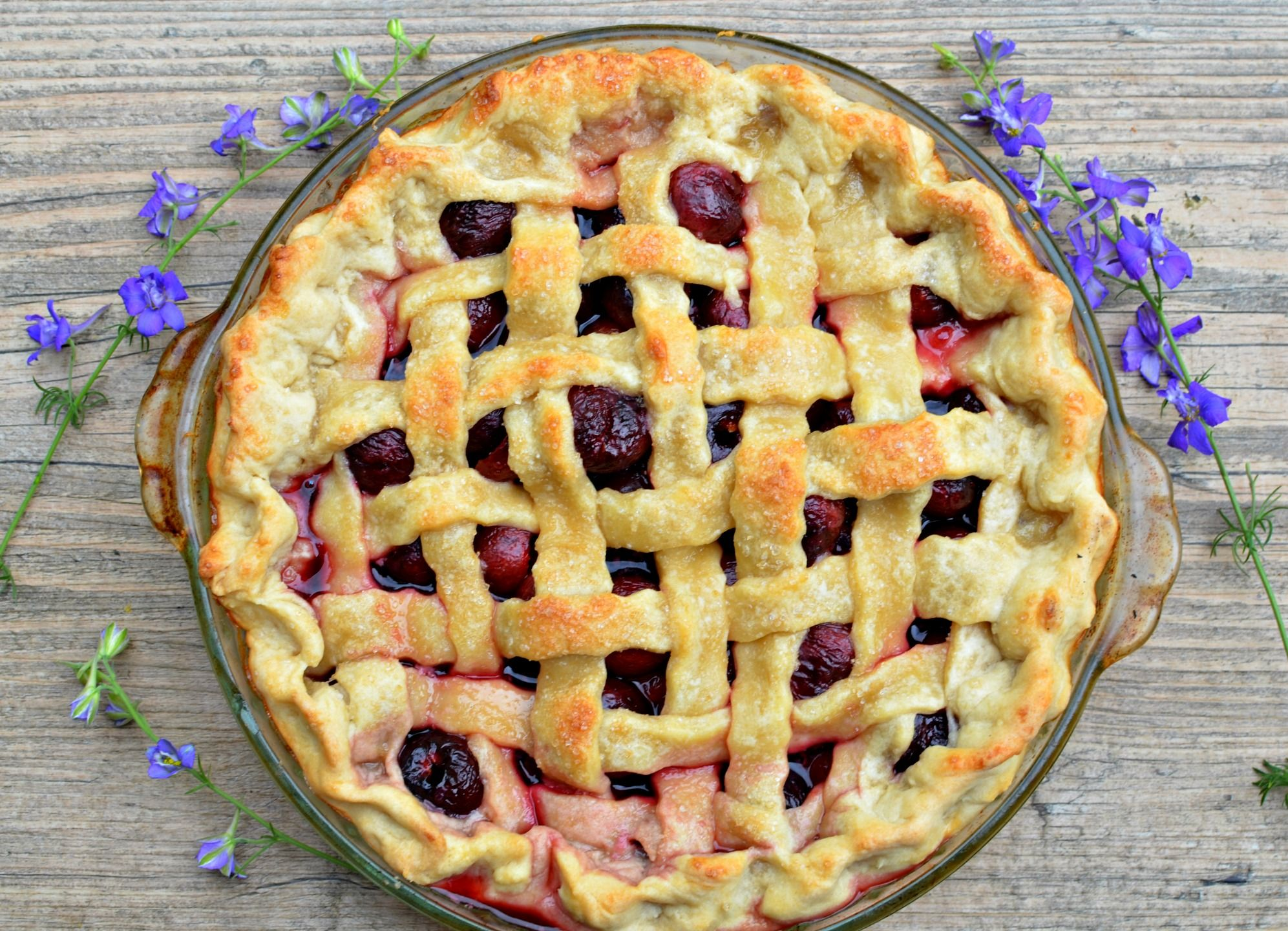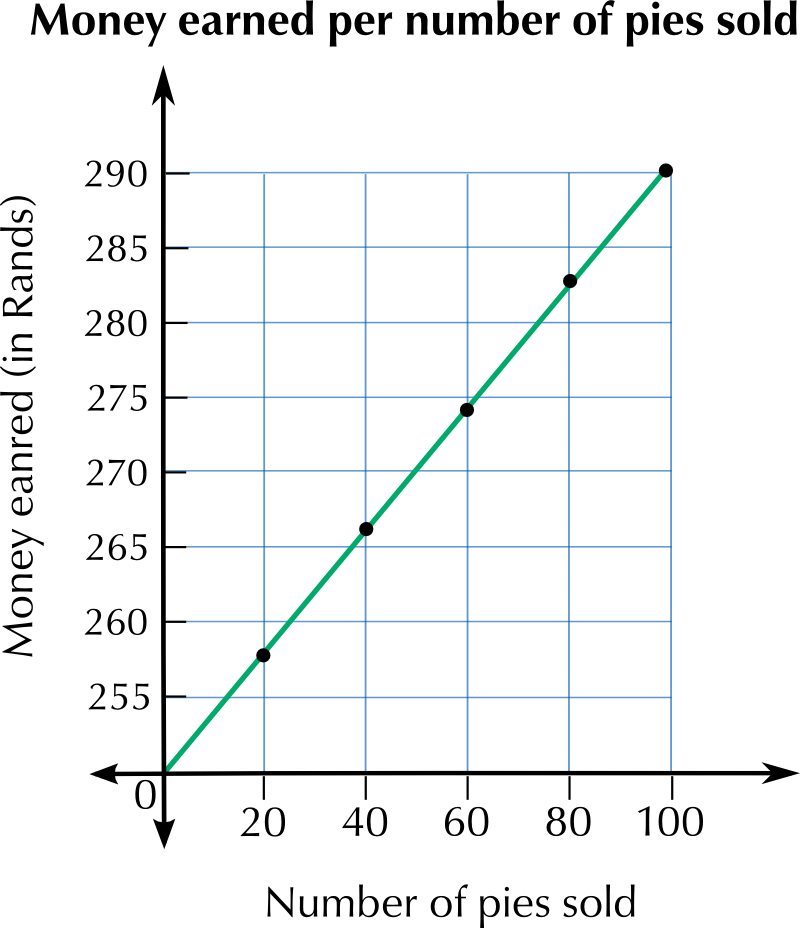Home Practice
For learners and parents For teachers and schools
Textbooks
Full catalogue
Pricing SupportLog in

We think you are located in United States. Is this correct?

# 2.5 Finding the rule or formula

## Worked example 6: Identifying patternsElvis makes boxes of fudge and works out the cost of making the boxes by using a pattern. Look at the table below.

 Number of boxes made $$\text{1}$$ $$\text{2}$$ $$\text{3}$$ $$\text{4}$$ $$\text{5}$$ $$\text{6}$$ Cost of making the boxes (R) $$\text{6}$$ $$\text{10}$$ $$\text{14}$$ $$\text{18}$$ $$\text{22}$$ $$\text{26}$$
1. What pattern do you see in this table?
2. Are there some different ways of describing the pattern?
3. What would it cost to make $$\text{20}$$ boxes of fudge? How do you know?
1. The cost increases by $$\text{R}\,\text{4}$$ as the number of boxes increases by $$\text{1}$$.
2. We could also say that the cost is equal to $$\text{2}$$, plus $$\text{4}$$ times the number of boxes. Or cost = $$\text{2}$$ + ($$\text{4}$$ $$\times$$ number of boxes).
3. From our answer to Question 2, we know that the cost is equal to $$\text{2}$$ plus $$\text{4}$$ times the number of boxes. $$\therefore$$ Cost = $$\text{2}$$ + ($$\text{4} \times \text{20}$$) = $$\text{2}$$ + $$\text{80}$$ = $$\text{82}$$ So it would cost $$\text{R}\,\text{82}$$ to make $$\text{20}$$ boxes.

In the previous example we described the cost pattern using the number of boxes. This is a very useful thing to do, because it gives us a rule that we can use for any number of boxes!

The rule written in words is: $$\text{cost }= \text{2} + (\text{4} \times \text{ number of boxes})$$

If we use variables to write the rule, we have:

$$c = \text{2} + \text{4}b$$, where $$c$$ = cost and $$b$$ = number of boxes.

Notice that in the pattern in the example above, the cost of making the boxes of fudge depends on the number of boxes made. So the cost ($$c$$) is the dependent variable. The number of boxes ($$b$$) is the independent variable.

### Writing a general formula (EMG3K)

Let's call the position of a number in a term $$n$$, so that we can use it to describe the value of the term. We call $$n$$ a variable, as it can represent different values.

A general formula for any term in the sequence in the table is $$(\text{10}n) - \text{5}$$.

(Remember that $$\text{10} \times n$$ can also be written as $$\text{10}n$$.)

So for the 100th term in this sequence, $$n = \text{100}$$ and the value of the term is $$(\text{10} \times \text{100}) - \text{5} = \text{995}$$.

What if you wrote a different number sentence for the pattern? You might have written:

$$\text{5} + [($$$$\text{1}$$$$- \text{1}) \times \text{10}] = \text{5} + \text{0} = \text{5}$$

$$\text{5} + [($$$$\text{2}$$$$- \text{1}) \times \text{10}] = \text{5} + \text{10} = \text{15}$$

$$\text{5} + [($$$$\text{3}$$$$- \text{1}) \times \text{10}] = \text{5} + \text{20} = \text{25}$$

If you replace the number in bold by $$n$$, you will get

$$\text{5} + [(n - \text{1}) \times \text{10}]$$. You will find that this simplifies as follows:

$$\text{5} + [(n - \text{1}) \times \text{10}]$$

$$= \text{5} + \text{10}n - \text{10}$$

## Describing patterns

Exercise 2.4

Describe each of these patterns in words, and then write three more terms in each sequence:

$$\text{2}$$; $$\text{4}$$; $$\text{8}$$; $$\text{16}$$;$$\ldots$$

This number sequence starts at $$\text{2}$$ and each term is multiplied by $$\text{2}$$ to get the next term.

$$\text{1}$$; $$\text{5}$$; $$\text{9}$$; $$\text{13}$$;$$\ldots$$

This number sequence starts at $$\text{1}$$ and $$\text{4}$$ is added to each term to get the next term.

$$\text{3}$$; $$\text{6}$$; $$\text{9}$$; $$\text{12}$$;$$\ldots$$

This number sequence starts at $$\text{3}$$ and $$\text{3}$$ is added to each term to get the next term.

$$\text{5}$$; $$\text{10}$$; $$\text{15}$$; $$\text{20}$$;$$\ldots$$

This number sequence starts at $$\text{5}$$ and $$\text{5}$$ is added to each term to get the next term.

Write down the first four terms of the pattern for each of the following descriptions:

This number sequence starts at $$\text{1}$$ and $$\text{20}$$ is added each time to get the next term.

$$\text{1}$$; $$\text{21}$$; $$\text{41}$$; $$\text{61}$$;$$\ldots$$

This number sequence starts at $$\text{1}$$ and each term is multiplied by $$\text{4}$$ to get the next term.

$$\text{1}$$; $$\text{4}$$; $$\text{16}$$; $$\text{64}$$;$$\ldots$$

This number sequence starts at $$\text{20 000}$$ and each term is multiplied by $$\text{2}$$ to get the next term.

$$\text{20 000}$$; $$\text{40 000}$$; $$\text{80 000}$$; $$\text{160 000}$$;$$\ldots$$

Complete the table for the following sequence and use the information to work out the general formula and the value of the 20th term: $$\text{5}$$; $$\text{14}$$; $$\text{23}$$; $$\text{32}$$; $$\text{41}$$; $$\text{50}$$;$$\ldots$$

 Position of term ($$n$$) $$\text{1}$$ $$\text{6}$$ $$\text{20}$$ Value of term $$\text{5}$$ $$\text{14}$$ $$\text{32}$$ $$\text{41}$$ $$\text{50}$$
 Position of term (n) $$\text{1}$$ $$\text{2}$$ $$\text{4}$$ $$\text{5}$$ $$\text{6}$$ $$\text{20}$$ Value of term $$\text{5}$$ $$\text{14}$$ $$\text{32}$$ $$\text{41}$$ $$\text{50}$$ $$\text{174}$$

Number sentence: $$(n \times \text{9}) - \text{4}$$. So 20th term $$=(\text{20} \times \text{9}) - \text{4} = \text{176}$$.

Kepa sells pies at a roadside stall. He earns a basic salary of $$\text{R}\,\text{250}$$ per day and a commission of $$\text{40}$$ $$\text{c}$$ on each pie he sells.Write an equation for calculating how much he earns at an event.
Earnings = $$\text{R}\,\text{250}$$ + ($$\text{40}\text{ c} \times n$$), where $$n$$ is number of pies he sells
Use your equation to complete the table:
 Number of pies $$\text{20}$$ $$\text{40}$$ $$\text{60}$$ $$\text{80}$$ $$\text{100}$$ Money earned (R)
 Number of pies $$\text{20}$$ $$\text{40}$$ $$\text{60}$$ $$\text{80}$$ $$\text{100}$$ Money earned (R) $$\text{258}$$ $$\text{266}$$ $$\text{274}$$ $$\text{282}$$ $$\text{290}$$
Plot the data points from your table and draw a graph.Should Kepa use the table, the graph or the equation to work out how much money he earns at the end of the day? Explain your answer.

Because the graph is continuous, it will allow him to calculate how much money he earned for any number of pies. The precision with which he can read off the graph however, depends on the intervals between units on the graph's axes.